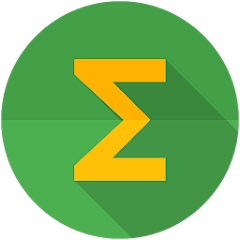# Math Formulas FREE

50K+Everyone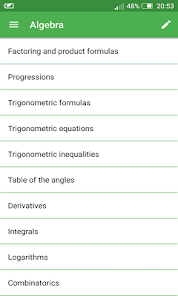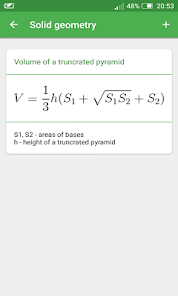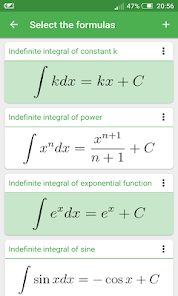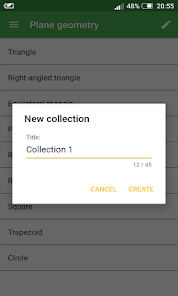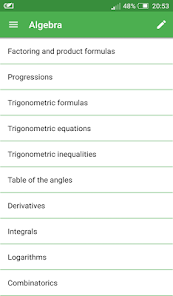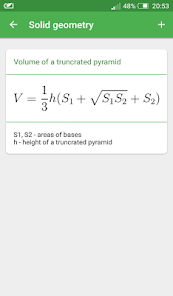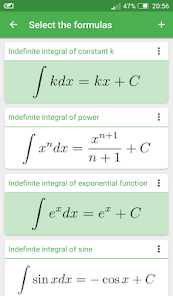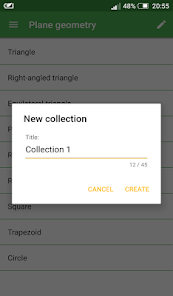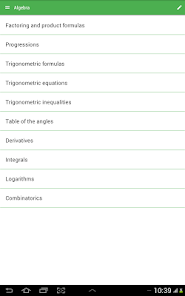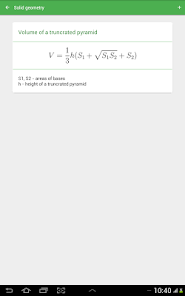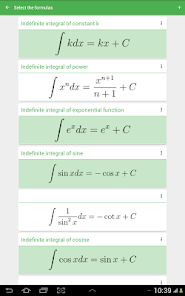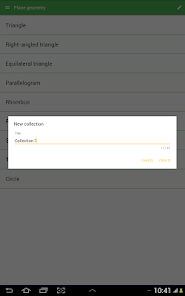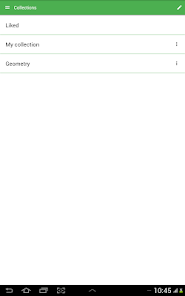The App “Math Formulas” is the best collection of mathematical formulas for education.

In the app Math Formulas you will find Algebra formulas, Plane geometry formulas, geometry formulas, Solid geometry formulas, trigonometry formulas and much more to help young students to learn this amazing and mysterious science. There is math formulas for all classes.

The App Math Formulas covers topics like:

• Factoring and product formulas
• Progressions
• Trigonometric formulas
• Trigonometric equations
• Trigonometric inequalities
• Table of the angles
• Derivatives
• Integrals
• Logarithms
• Combinatorics
• Triangle
• Right-angled triangle
• Equilateral triangle
• Parallelogram
• Rhombus
• Rectangle
• Square
• Trapezoid
• Circle
• Coordinates and vectors
• Cube
• Prism
• Parallelepiped
• Pyramid
• Regular pyramid
• Truncated pyramid
• Cylinder
• Cone
• Frustum
• Sphere and spherical sector

Features:

• Intuition and friendliness material UI
• Working without internet connection.
• Plurality of the formulas

All essentials math formulas and equations in one app - that's great!
Updated on
May 16, 2017

## Data safety